Download Presentation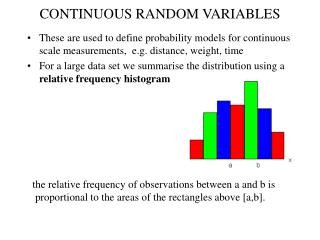CONTINUOUS RANDOM VARIABLES

# CONTINUOUS RANDOM VARIABLES - PowerPoint PPT Presentation

CONTINUOUS RANDOM VARIABLES. These are used to define probability models for continuous scale measurements, e.g. distance, weight, time For a large data set we summarise the distribution using a relative frequency histogram. the relative frequency of observations between a and b isI am the owner, or an agent authorized to act on behalf of the owner, of the copyrighted work described.
Download Presentation## CONTINUOUS RANDOM VARIABLES

An Image/Link below is provided (as is) to download presentation

Download Policy: Content on the Website is provided to you AS IS for your information and personal use and may not be sold / licensed / shared on other websites without getting consent from its author.While downloading, if for some reason you are not able to download a presentation, the publisher may have deleted the file from their server.

- - - - - - - - - - - - - - - - - - - - - - - - - - E N D - - - - - - - - - - - - - - - - - - - - - - - - - -
Presentation Transcript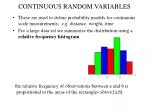CONTINUOUS RANDOM VARIABLES
• These are used to define probability models for continuous scale measurements, e.g. distance, weight, time
• For a large data set we summarise the distribution using a relative frequency histogram

the relative frequency of observations between a and b is

proportional to the areas of the rectangles above [a,b].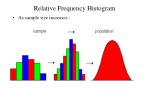Relative Frequency Histogram

• As sample size increases :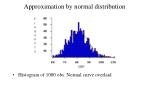Approximation by normal distribution
• Histogram of 1000 obs. Normal curve overlaid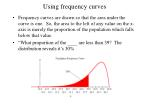Using frequency curves
• Frequency curves are drawn so that the area under the curve is one. So, the area to the left of any value on the x-axis is merely the proportion of the population which falls below that value.
• “What proportion of the ____ are less than 39? The distribution reveals it’s 30%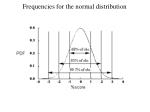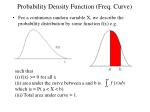Probability Density Function (Freq. Curve)
• For a continuous random variable X, we describe the probability distribution by some function f(x) e.g.

such that

(i) f(x) >= 0 for all x

(ii) area under the curve between a and b is

which is = P( a < X < b)

(iii) Total area under curve = 1.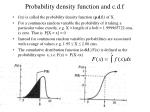Probability density function and c.d.f
• f(x) is called the probability density function (p.d.f.) of X.
• For a continuous random variable the probability of it taking a particular value exactly, e.g. X = length of a bolt = 1.999965722 cms, is zero. That is P[X = x] = 0
• Instead for continuous random variables probabilities are associated with a range of values.e.g. 1.95  X  2.00 cms.
• The cumulative distribution function (c.d.f.) F(x) is defined as the probability upto x, i.e. F(x) = P(X <x)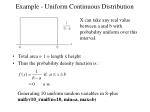Example - Uniform Continuous Distribution
• Total area = 1 = length x height
• Thus the probability density function is :

X can take any real value between a and b with probability uniform over this interval.

Generating 10 uniform random variables in S-plus

unifrv10_runif(n=10, min=a, max=b)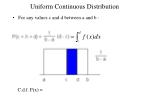Uniform Continuous Distribution
• For any values c and d between a and b :

C.d.f. F(x) =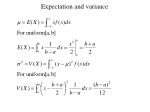Normal distributions
• Normal distributions are one type of continuous p.d.f.
• If X has the Normal distribution with mean µ and variance 2, this is denoted by X~N(µ,2) (Splus uses s.d. instead of var)
• Z ~ N(µ=0,2=1) is called the standard normal distribution
• Since normal probabilities are hard to compute, tables were made for the standard normal distribution only
• Most textbooks give areas under the curve of the N(0,1) p.d.fCalculating standard normal probabilities

Find the probability of getting a value of Z greater than 1.05

P(Z>1.05)= 1 - P(Z<1.05)

look up P(Z<1.05) in tables

P(Z<1.05) = 0.8531

P(Z>1.05)=

Find the prob of Z between -1.05 and 1.05

P(-1.05<Z<1.05) =

P(Z<1.05) - P(Z<-1.05)

= 0.8531 - P(Z>1.05)

=In order to obtain probabilities for other Normal distributions (i.e. areas under the curve), it is necessary to express any value of X in terms of the number of standard deviation units it is away from µ.Example of normal distribution
• A filling machine is used to fill soft drink bottles. The bottles are supposed to contain 300 mls. In fact the quantities vary according to the Normal distribution with expected value of µ = 302 ml and standard deviation s = 3ml. What is the probability that an individual bottle contains less than 295 mls?

Let the r.v. X denote the quantity in an individual bottle. We are told X ~ N(302, 32), and we want Pr{X < 295}.

If X = 295 then Z = (295 - 302)/3 = -2.33

so P(X < 295) = P(Z < -2.33) = 1 - P(Z < +2.33)

= 1 - .990 = 0.01

i.e. about 1 bottle in 100 would have less than 295 ml.Normal probabilities from R
• e.g. If X ~ N(5,9)

(i) find P(X < 7)

(ii) find k such that P(X < k) = 0.05

• p7_pnorm(q=7, mean=5, sd=3) (0.7475)
• q0.05_qnorm(p=0.05, mean=5, sd=3) (0.0654)
• Check using tablesExponential distribution
• T = time to first arrival
• P(T > t) = P(N(t) = 0) =(lt)0 e-lt / 0! = e-lt
• c.d.f of T FT(t) = P(T< t) = 1 - e-lt
• p.d.f. of T fT(t)= d/dt FT(t) = le-lt

E(T) = 1/l

V(T) = 1/l2Residual time distribution

T

t

R

P(R>r) =P(T>r+t |T>t)

=P(T>r+t)/P(T>t)

= e-(r+t) /e-t = = e-r

= P (T > r)

time up to next arrival is independent of when the previous arrival occurred

• Distr of additionallifetime is same as the original distr.
• Memoryless property of exponential
• Other distributions such as gamma, Weibull etc. are not memoryless (exponential is the only one).Quantiles
• Cumulative distribution function F(x):
• F(x) = P( X < x)
• e.g. Z ~ N(0,1) P(Z < 1.96) = ?
• need to look at the c.d.f. curve to answer this question
• F(1.96) = 0.975 P(Z < 1.96) = 0.975
• i.e. 1.96 is the 97.5 th percentile of N(0,1). 1.96 = F-1(0.975)
• What is the 50th percentile of N(0,1) ?
• What is the 60th percentile of N(0,1) ?
• Ans: Look at quantile plot.
• Quantile plot: x-axis - cumulative probability (0,1) y-axis: F-1
• Quantile plot is inverse of c.d.f. plot.